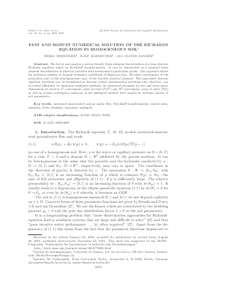Repository: Freie Universität Berlin, Math Department

# Fast and robust numerical solution of the Richards equation in homogeneous soil

Berninger, H. and Kornhuber, R. and Sander, O. (2011) Fast and robust numerical solution of the Richards equation in homogeneous soil. SIAM Journal on Numerical Analysis, 49 (6). pp. 2576-2597. ISSN 0036-1429Preview

2MB

Official URL: http://dx.doi.org/10.1137/100782887

## Abstract

We derive and analyze a solver-friendly finite element discretization of a time discrete Richards equation based on Kirchhoff transformation. It can be interpreted as a classical finite element discretization in physical variables with nonstandard quadrature points. Our approach allows for nonlinear outflow or seepage boundary conditions of Signorini type. We show convergence of the saturation and, in the nondegenerate case, of the discrete physical pressure. The associated discrete algebraic problems can be formulated as discrete convex minimization problems and, therefore, can be solved efficiently by monotone multigrid methods. In numerical examples for two and three space dimensions we observe $L^2$-convergence rates of order $\mathcal{O}(h^2)$ and $H^1$-convergence rates of order $\mathcal{O}(h)$ as well as robust convergence behavior of the multigrid method with respect to extreme choices of soil parameters.

Item Type: Article Saturated-unsaturated porous media flow, Kirchhoff transformation, convex minimization, finite elements, monotone multigrid Mathematical and Computer Sciences > Mathematics > Numerical Analysis Department of Mathematics and Computer Science > Institute of Mathematics 1799 Ekaterina Engel 18 Feb 2016 09:53 03 Mar 2017 14:41

Repository Staff Only: item control page